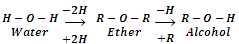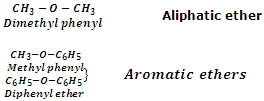What are Ethers and its types?

Ethers are the compounds with general formula or CnH2n+2O (same as monohydric alcohols). These are symbolized through a general structure. R-O-R'. They may be regarded as dialkyl derivatives of water or mono alkyl derivatives of alcohols.They are also considered as anhydrides of alcohols because they can be obtained by the elimination of water molecule from two alcohol molecules.The groups R and R' in ether may either be same or different. In case these groups are same, the compounds are known as simple ethers or symmetrical ethers. On the other hand, if R and R' groups are different, the compounds are called mixed ether or unsymmetrical ethers.

C2H5-O-C2H5      Simple ether

C2H5-O-CH3         Mixed ether

Ethers may also be classified into two categories as aliphatic ethers and aromatic ethers. In aliphatic ethers, the groups R and R' are both alkyl groups whereas in aromatic ethers, one or both R and R' groups are aryl groups. Ether with one alkyl and one aryl groups are also called aryl alkyl ethers or phenolic ethers whereas ethers with both aryl groups are called diaryl ethers. Few examples are taken below:#### Related Questions in Chemistry

• ##### Q :Determining of normality of sodium

Can someone please help me in getting through this problem. The normality of a solution of sodium hydroxide 100 ml of which includes 4 grams of NaOH is: (a) 0.1 (b) 40 (c) 1.0 (d) 0.4

• ##### Q :Molarity of solution Help me to go

Help me to go through this problem. When 7.1gm Na2SO4 (molecular mass 142) dissolves in 100ml H2O , the molarity of the solution is: (a) 2.0 M (b) 1.0 M (c) 0.5 M (d) 0.05 M

• ##### Q :Production of alcoholic drinks give all

give all physical aspects in the production of alcohol

• ##### Q :Means of molality Give me answer of

Give me answer of this question. The number of moles of solute per kg of a solvent is called its: (a) Molarity (b) Normality (c) Molar fraction (d) Molality

• ##### Q :Relative lowering of the vapour pressure

Choose the right answer from following.The relative lowering of the vapour pressure is equal to the ratio between the number of: (a) Solute moleules and solvent molecules (b) Solute molecules and the total molecules in the solution (c) Solvent molecules and the tota

• ##### Q :Unit of mole fraction Provide solution

Provide solution of this question. Unit of mole fraction is: (a) Moles/litre (b) Moles/litre2 (c) Moles-litre (d) Dimensionless

• ##### Q :Problems related to entropy change A)

A) Two compartments each of 1 m3 capacity are joined by a valve and insulated from the surroundings and from one another. One compartment has saturated steam at 683.6 kPa and the other contains steam at the same temperature but at a pressure of 101.3 kPa. T

• ##### Q :Define alum Illustrate alum?

Illustrate alum?

• ##### Q :Product of HCl Zn Illustrate  the

Illustrate  the product of HCl Zn?

• ##### Q :Mole fraction Give me answer of

Give me answer of following question. The sum of the mole fraction of the components of a solution is : (a) 0 (b) 1 (c) 2 (d) 4.